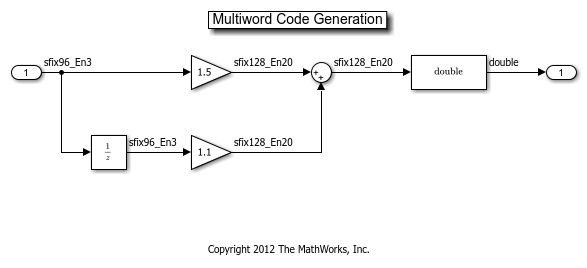# Fixed-Point Multiword Operations In Generated Code

This example shows how to control generation of multiword operations in generated code.

In this example you will learn:

• How to generate code for big data types using multiword operations

• How to prevent multiword code when it is not expected

### Simple Multiword Operation

This model shows how wide integer and fixed-point operations become multiword in the generated C code. Multiword code is normally triggered by using parameters or signals with data types wider than C 'long'.

```open_system('fxpdemo_multiword_example1'); set_param('fxpdemo_multiword_example1','SimulationCommand','Update'); ```Generate code for the model:

```evalc('slbuild(''fxpdemo_multiword_example1'');'); % Suppress output ```

In the generated code, multiword operations are implemented using functions. These functions will have "MultiWord" in their name.

Review one of the multiword functions that was generated: `MultiWordAdd()`

```fid = fopen('fxpdemo_multiword_example1_grt_rtw/fxpdemo_multiword_example1.c') ; ctext = fread(fid, '*char')'; fclose(fid); match = regexp(ctext, 'void MultiWordAdd.*?\n\}', 'match'); disp(match{1}); ```
```void MultiWordAdd(const uint32_T u1[], const uint32_T u2[], uint32_T y[], int32_T n) { int32_T i; uint32_T carry = 0U; uint32_T u1i; uint32_T yi; for (i = 0; i < n; i++) { u1i = u1[i]; yi = (u1i + u2[i]) + carry; y[i] = yi; carry = carry != 0U ? (uint32_T)(yi <= u1i) : (uint32_T)(yi < u1i); } } ```

This function implements multiword addition in C. The two operands and the result all have the same number of words, and the addition is performed one word at a time.

```close_system('fxpdemo_multiword_example1', 0); ```

### Relational Operator Block

In the Relational Operator Block example below, you would expect to get multiword functions in the generated code. The two input data types are `uint32` and `ufix32_En3`. A good type for comparison is `ufix35_En3`, because this type can represent all the real world values of both operands.

We expect the data type `ufix35_En3` to be implemented using a 64-bit two-word data type.

```open_system('fxpdemo_multiword_example2'); set_param('fxpdemo_multiword_example2','SimulationCommand','Update'); ```This model is configured for a CPU with 32-bit C type long. A 64-bit data type will be a multiword type.

```get_param(bdroot, 'ProdBitPerLong') ```
```ans = 32 ```

Generate code for the model and review:

```evalc('slbuild(''fxpdemo_multiword_example2'');'); % Suppress output fid = fopen('fxpdemo_multiword_example2_grt_rtw/fxpdemo_multiword_example2.c') ; ctext = fread(fid, '*char')'; fclose(fid); match = regexp(ctext, 'void fxpdemo_multiword_example2_step.*?\n\}', 'match'); disp(match{1}); ```
```void fxpdemo_multiword_example2_step(void) { /* RelationalOperator: '<Root>/LessThan' incorporates: * Inport: '<Root>/In1' * Inport: '<Root>/In2' */ Y = ((U1 <= 536870911U) && ((U1 << 3) < U2)); } ```

Multiword code was not generated. This code is single-word and uses a comparison data type of `uint32`. As a result, precision loss in the comparison may occur.

Simulink balances the requirements for the internal data type for comparison. In this case, because all data types are single word, it implements an efficient data type that produces small, fast code rather than a more precise, cumbersome computation.

```close_system('fxpdemo_multiword_example2', 0); ```

To improve the precision of this calculation, do one of the following steps:

• Pick input data types that can be compared with full precision using a single word comparison type. For example, 16-bit types, or two identical types.

• Force Simulink to use a multiword type (during both simulation and code generation) by specifying a multiword type for at least one of the inputs. This signals Simulink that you want to use multiword operations for this block.

• Configure the model for a 64-bit system.

### MATLAB Function Block

The MATLAB Function Block example below showcases an all-single-word calculation. Multiword code is not expected.

```open_system('fxpdemo_multiword_example3'); set_param('fxpdemo_multiword_example3','SimulationCommand','Update'); ``````mfb = get_param('fxpdemo_multiword_example3/MATLAB Function','MATLABFunctionConfiguration'); mfb.FunctionScript ```
```ans = 'function y = fcn(u1, u2) %#codegen y = fi(u1 * u2, 0, 32, 0);' ```

Generate code for the model:

```evalc('slbuild(''fxpdemo_multiword_example3'');'); % Suppress output fid = fopen('fxpdemo_multiword_example3_grt_rtw/fxpdemo_multiword_example3.c') ; ctext = fread(fid, '*char')'; fclose(fid); match = regexp(ctext, 'void fxpdemo_multiword_example3_step.*?\n\}', 'match'); disp(match{1}); ```
```void fxpdemo_multiword_example3_step(void) { uint64m_T tmp; uint64m_T tmp_0; /* MATLAB Function: '<Root>/MATLAB Function' incorporates: * Inport: '<Root>/In1' * Inport: '<Root>/In2' */ /* MATLAB Function 'MATLAB Function': '<S1>:1' */ /* '<S1>:1:4' */ uMultiWordMul(&U1, 1, &U2, 1, &tmp_0.chunks[0U], 2); uMultiWordShrNear(&tmp_0.chunks[0U], 2, 3U, &tmp.chunks[0U], 2); /* Outport: '<Root>/Out1' incorporates: * MATLAB Function: '<Root>/MATLAB Function' */ fxpdemo_multiword_example3_Y.Out1 = uMultiWord2uLongSat(&tmp.chunks[0U], 2); } ```
```close_system('fxpdemo_multiword_example3', 0); ```

Even though all the data types in the model were single-word, you still got three calls to multiword functions, and two multiword variables as well.

Fixed-point operations in the MATLAB Function Block are controlled by fimath property settings.

```fimath ```
```ans = RoundingMethod: Nearest OverflowAction: Saturate ProductMode: FullPrecision SumMode: FullPrecision ```

This fimath specifies full precision `ProductMode`. Therefore multiplications are performed in a way that preserves as much precision as possible. The product data type, which is `uint64_En3`, is implemented as a multiword type.

You can control multiword in code generation for MATLAB code by manipulating the fimath. For example:

• Adjusting fimath properties to meet efficient code requirements. In this example, set `'ProductMode'` to `'KeepLSB'` and `'OverflowAction'` to `'Wrap'`.

• Defining local fimaths in the MATLAB Function Block that are tailored for the specific calculations, and not relying on the global fimath.

```load_system('fxpdemo_multiword_example4'); % No need to show this model. Only show the MATLAB code. mfb = get_param('fxpdemo_multiword_example4/MATLAB Function','MATLABFunctionConfiguration'); mfb.FunctionScript ```
```ans = 'function y = fcn(u1, u2) %#codegen F = fimath('ProductMode','KeepLSB',... 'ProductWordLength',32,... 'OverflowAction','Wrap'); u1 = setfimath(u1,F); u2 = setfimath(u2,F); y = fi(u1 * u2,0,32,0); ' ```

This fimath will result in this generated code:

```evalc('slbuild(''fxpdemo_multiword_example4'');'); % Suppress output fid = fopen('fxpdemo_multiword_example4_grt_rtw/fxpdemo_multiword_example4.c') ; ctext = fread(fid, '*char')'; fclose(fid); match = regexp(ctext, 'void fxpdemo_multiword_example4_step.*?\n\}', 'match'); disp(match{1}); ```
```void fxpdemo_multiword_example4_step(void) { uint32_T tmp; /* MATLAB Function: '<Root>/MATLAB Function' incorporates: * Inport: '<Root>/In1' * Inport: '<Root>/In2' */ /* MATLAB Function 'MATLAB Function': '<S1>:1' */ /* '<S1>:1:6' */ /* '<S1>:1:7' */ /* '<S1>:1:8' */ tmp = U1 * U2; Y1 = (uint32_T)((tmp & 4U) != 0U) + (tmp >> 3); } ```
```close_system('fxpdemo_multiword_example4', 0); ```
```clear ctext fid match mfb clear ans ```